# Breakpoint analysis, segmented regression

With some side-notes on regression, polynoms, curve-fitting

An introduction to the package “segmented”: Segmented relationships in regression models with breakpoints/changepoints estimation

http://cran.r-project.org/web/packages/segmented/index.html

``````# breakpoint analysis, segmented regression
# assuming segments are continuous! That means, start of a segment is at the same position as the end of the previous segment.

library(ggplot2)

setwd("~/Prac/R_demo/breakpoint")

# import data
# those data are from a recent bike ride in the Alps from Mt Beauty to Falls Creek.
# Data are from my GPS-receiver.

# create a figure to get an idea of the data
p <- ggplot(df, aes(x = DistanceMeters, y = AltitudeMeters)) + geom_line()
p
``````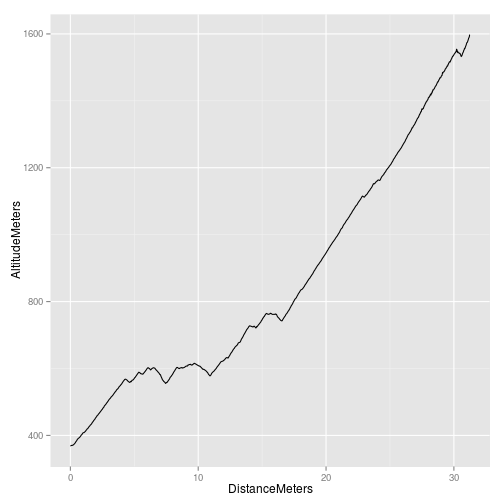``````
p <- p + labs(x = "Distance [km]",
y = "Elevation above sea level [m]")
p
``````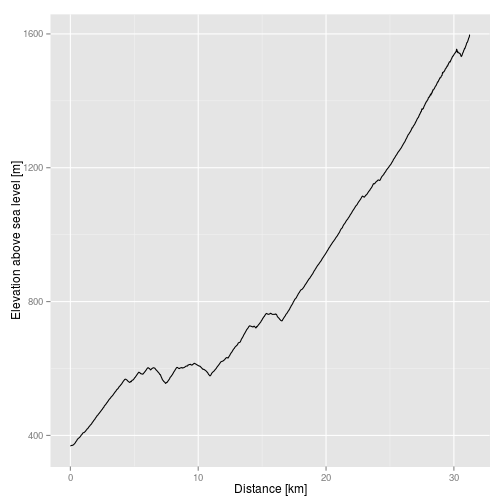``````
# create a linear model
my.lm <- lm(AltitudeMeters ~ DistanceMeters, data = df)
summary(my.lm)
``````
``````##
## Call:
## lm(formula = AltitudeMeters ~ DistanceMeters, data = df)
##
## Residuals:
##     Min      1Q  Median      3Q     Max
## -157.02  -88.66   -7.81   89.50  153.17
##
## Coefficients:
##                Estimate Std. Error t value Pr(>|t|)
## (Intercept)    285.5073     1.8355     156   <2e-16 ***
## DistanceMeters  37.0932     0.0958     387   <2e-16 ***
## ---
## Signif. codes:  0 '***' 0.001 '**' 0.01 '*' 0.05 '.' 0.1 ' ' 1
##
## Residual standard error: 88.9 on 10310 degrees of freedom
## Multiple R-squared:  0.936,  Adjusted R-squared:  0.936
## F-statistic: 1.5e+05 on 1 and 10310 DF,  p-value: <2e-16
``````

The ride starts steep, flattens out with some undulations and ends with a long but consistent ascent. A linear regression of the last part is quite different from other parts of the data. For example:

``````# a linear model with data for the part after 16 km
my.lm2 <- lm(AltitudeMeters ~ DistanceMeters, data = df[df\$DistanceMeters > 16, ])
summary(my.lm2)
``````
``````##
## Call:
## lm(formula = AltitudeMeters ~ DistanceMeters, data = df[df\$DistanceMeters >
##     16, ])
##
## Residuals:
##    Min     1Q Median     3Q    Max
## -22.84  -6.66   1.43   7.06  46.73
##
## Coefficients:
##                Estimate Std. Error t value Pr(>|t|)
## (Intercept)    -199.644      0.876    -228   <2e-16 ***
## DistanceMeters   57.154      0.036    1585   <2e-16 ***
## ---
## Signif. codes:  0 '***' 0.001 '**' 0.01 '*' 0.05 '.' 0.1 ' ' 1
##
## Residual standard error: 12.1 on 5766 degrees of freedom
## Multiple R-squared:  0.998,  Adjusted R-squared:  0.998
## F-statistic: 2.51e+06 on 1 and 5766 DF,  p-value: <2e-16
``````
``````
# Extract te coefficients from the overall model
my.coef <- coef(my.lm)

# add the regression line to the graph
# setting the aesthetics to a constant - this provides a name that we can reference later when we add additional layers
p <- p + geom_abline(intercept = my.coef,
slope = my.coef,
aes(colour = "overall"))
p
``````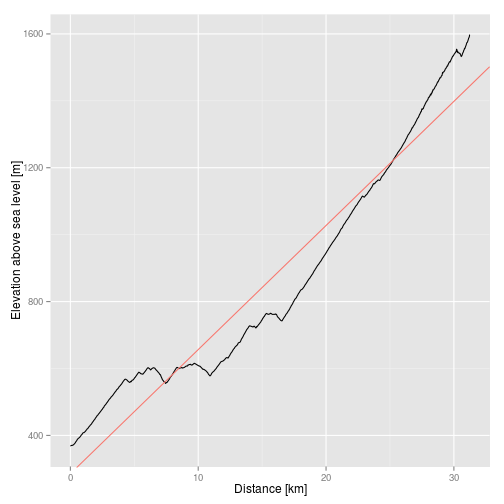Some notes on regression that we did not cover previously

``````#for fun: Polynom, third degree: ?poly
# how to use a polynom in a linear model
my.lm3 <- lm(AltitudeMeters ~ poly(DistanceMeters, 3), data = df)

p + geom_smooth(method = "lm",
formula = y ~ poly(x, degree = 3),
se = FALSE, colour = "orange")
``````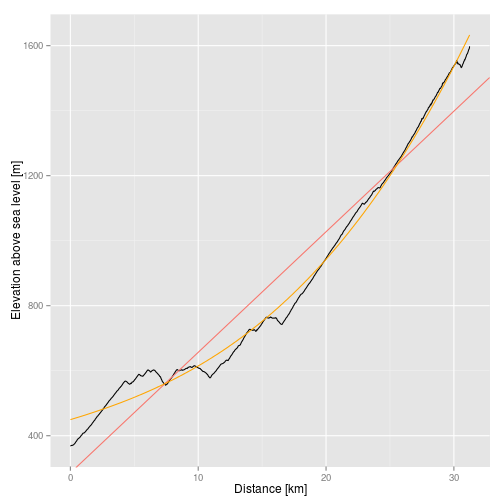A general overview on frequently used curves/equations for curve fitting is available from:

http://www.for.gov.bc.ca/hfd/pubs/docs/bio/bio04.htm (Thanks RD for that - still helpful today)

``````
# More regression remarks
# regression through the origin: see ?lm
my.lm2 <- lm(AltitudeMeters ~ DistanceMeters - 1, data = df)
my.coef2 <- coef(my.lm2)

# regression with a slope of 1, see ?offset
my.lm3 <- lm(AltitudeMeters ~ offset(DistanceMeters), data = df)
my.coef3 <- coef(my.lm3)
``````

## Now for the actual break point analysis

``````# -------------------
# analyse breakpoints
# -------------------
# http://cran.r-project.org/doc/Rnews/Rnews_2008-1.pdf
library(segmented)

# have to provide estimates for breakpoints.
# after looking a the data,
my.seg <- segmented(my.lm,
seg.Z = ~ DistanceMeters,
psi = list(DistanceMeters = c(4, 15)))

# When not providing estimates for the breakpoints "psi = NA" can be used.
# The number of breakpoints that will show up is not defined
#my.seg <- segmented(my.lm,
#                    seg.Z = ~ DistanceMeters,
#                    psi = NA)

# display the summary
summary(my.seg)
``````
``````##
##  ***Regression Model with Segmented Relationship(s)***
##
## Call:
## segmented.lm(obj = my.lm, seg.Z = ~DistanceMeters, psi = list(DistanceMeters = c(4,
##     15)))
##
## Estimated Break-Point(s):
##                        Est.  St.Err
## psi1.DistanceMeters  3.527 0.03871
## psi2.DistanceMeters 16.330 0.01957
##
## t value for the gap-variable(s) V:  0 0
##
## Meaningful coefficients of the linear terms:
##                   Estimate Std. Error t value Pr(>|t|)
## (Intercept)        359.111      1.234   291.1   <2e-16 ***
## DistanceMeters      48.119      0.569    84.5   <2e-16 ***
## U1.DistanceMeters  -32.268      0.576   -56.1       NA
## U2.DistanceMeters   41.484      0.103   404.4       NA
## ---
## Signif. codes:  0 '***' 0.001 '**' 0.01 '*' 0.05 '.' 0.1 ' ' 1
##
## Residual standard error: 18.8 on 10306 degrees of freedom
## Multiple R-Squared: 0.997,  Adjusted R-squared: 0.997
##
## Convergence attained in 6 iterations with relative change -9.592e-06
``````
``````
# get the breakpoints
my.seg\$psi
``````
``````##                     Initial   Est.  St.Err
## psi1.DistanceMeters       4  3.527 0.03871
## psi2.DistanceMeters      15 16.328 0.01957
``````
``````
# get the slopes
slope(my.seg)
``````
``````## \$DistanceMeters
##        Est. St.Err. t value CI(95%).l CI(95%).u
## slope1 48.1  0.5690    84.5      47.0      49.2
## slope2 15.9  0.0850   186.0      15.7      16.0
## slope3 57.3  0.0574   999.0      57.2      57.4
``````
``````
# get the fitted data
my.fitted <- fitted(my.seg)
my.model <- data.frame(Distance = df\$DistanceMeters, Elevation = my.fitted)

# plot the fitted model
ggplot(my.model, aes(x = Distance, y = Elevation)) + geom_line()
``````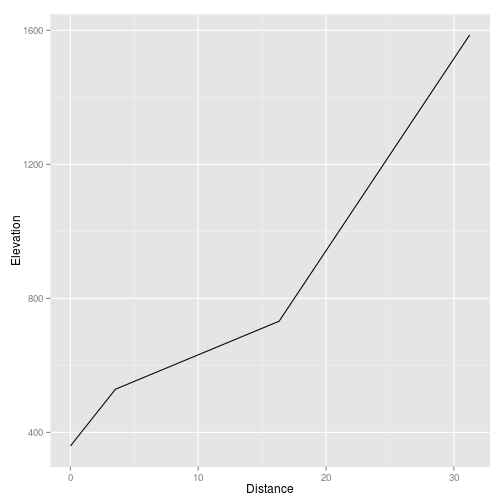``````
# add the fitted data to the exisiting plot
p + geom_line(data = my.model, aes(x = Distance, y = Elevation), colour = "tomato")
``````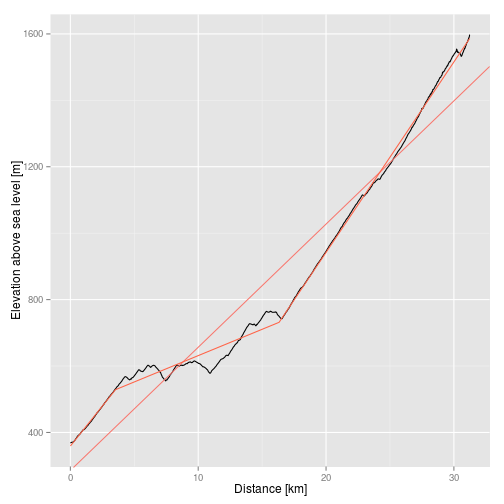``````

# add vertical lines to indicate the break locations
# second row of the psi-matrix
my.lines <- my.seg\$psi[, 2]

p <- p + geom_vline(xintercept = my.lines, linetype = "dashed")
p
``````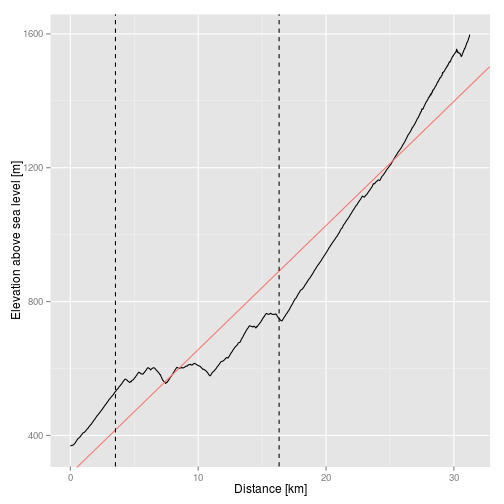``````

# get the slopes manually - excercise!!
my.slopes <- coef(my.seg)

# first line:
#y = b0 + b1*x
#y = intercept1 + slope1 * x

# second line:
#y = c0 + c1*x
#y = intercept2 + slope2 * x

# third line
#y = d0 + d1 *x
#y = intercept3 + slope3 * x

# At the breakpoint (break1), the segments b and c intersect

#b0 + b1*x = c0 + c1*x

b0 <- coef(my.seg)[]
b1 <- coef(my.seg)[]

# Important:
# the coefficients are the differences in slope in comparison to the previous slope
c1 <- coef(my.seg)[] + coef(my.seg)[]
break1 <- my.seg\$psi[]

#Solve for c0 (intercept of second segment):
c0 <- b0 + b1 * break1 - c1 * break1

# At the breakpoint (break2), the two lines are the same again:
# the coefficients are the differences in slope in comparison to the previous slope
d1 <- coef(my.seg)[] + c1
break2 <- my.seg\$psi[]

#Solve for d0 (intercept of third segment):
d0 <- c0 + c1 * break2 - d1 * break2

# adding lines to the graph

# line before first breakpoint
p <- p + geom_abline(intercept = b0, slope = b1,
aes(colour = "first part"), show_guide = TRUE)
p
``````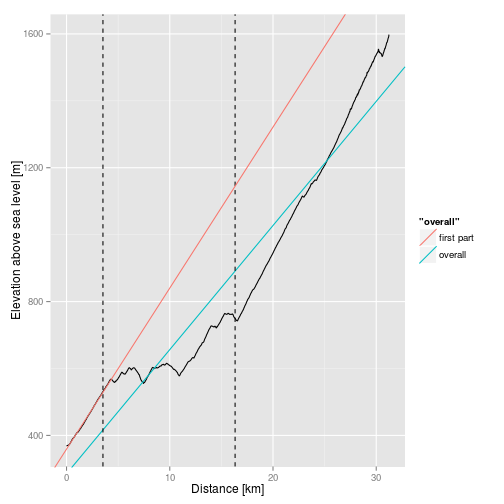``````
# line after first breakpoint
p <- p + geom_abline(intercept = c0, slope = c1,
aes(colour = "second part"), show_guide = TRUE)
p
``````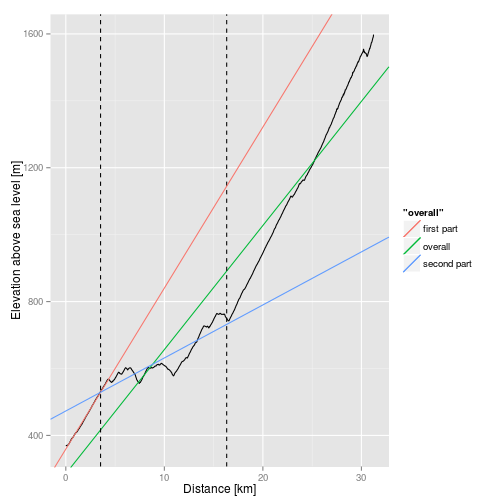``````
# line after second breakpoint
p <- p + geom_abline(intercept = d0, slope = d1,
aes(colour = "third part"), show_guide = TRUE)
p
``````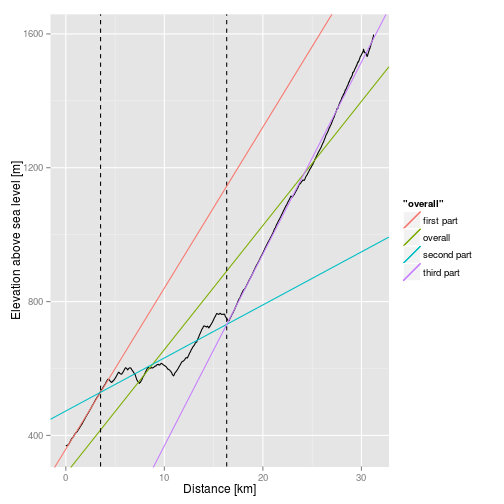``````
# create a manual colour scale, based on the previously assigned aesthetics,
# have to specify the "breaks" manually to achieve a specific, non-alphabetic order
p <- p + scale_colour_manual(name = "Lines",
values = c("overall"     = "red",
"first part"  = "blue",
"second part" = "green",
"third part"  = "yellow"),
breaks = c("overall", "first part", "second part", "third part"))
p
``````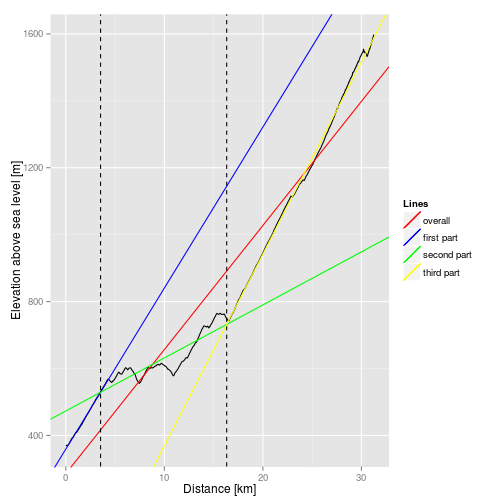``````
# And for fun, the ride followed a fourth-order polynom:
p + geom_smooth(method = "lm",
formula = y ~ poly(x, 4), se = FALSE, colour = "brown")
``````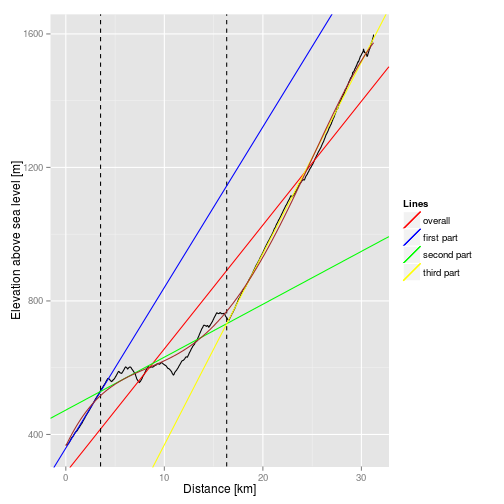See package “strucchange” for breakpoint analysis when segments are not required to join.